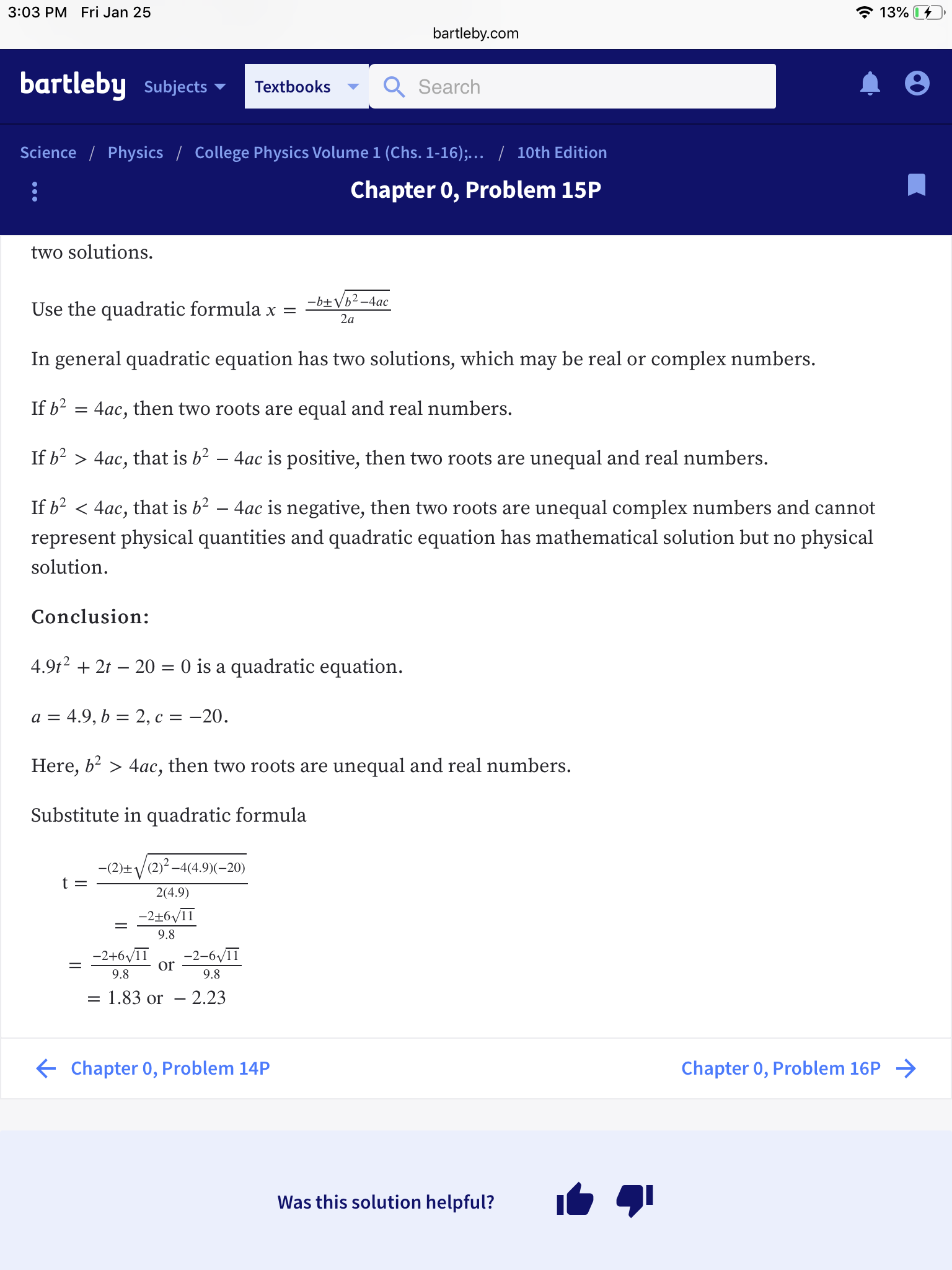# 3:03 PM Fri Jan 25 139 bartleby.com bartleby subjects Search Textbooks Science Physics College Physics Volume 1 (Chs. 1-16), 10th Edition Chapter 0, Problem 15P two solutions. Use the quadratic formula x = In general quadratic equation has two solutions, which may be real or complex numbers. If b2 - 4ac, then two roots are equal and real numbers. If b2 〉 4ac, that is b2-4ac is positive, then two roots are unequal and real numbers. If b2く4ac, that is b2-4ac is negative, then two roots are unequal complex numbers and cannot represent physical quantities and quadratic equation has mathematical solution but no physical solution Conclusion: 4.9t2 + 2t -20- 0 is a quadratic equation Here, b- > 4ac, then two roots are unequal and real numbers. Substitute in quadratic formula 2(4.9) 9.8 -2+6/1-2-6/11 Or 9.8 9.8 1.83 or - 2.23 Chapter 0, Problem 14P Chapter 0, Problem 16P Was this solution helpful?

Question

I need help understanding how they got 6 square root of 11.help_outlineImage Transcriptionclose3:03 PM Fri Jan 25 139 bartleby.com bartleby subjects Search Textbooks Science Physics College Physics Volume 1 (Chs. 1-16), 10th Edition Chapter 0, Problem 15P two solutions. Use the quadratic formula x = In general quadratic equation has two solutions, which may be real or complex numbers. If b2 - 4ac, then two roots are equal and real numbers. If b2 〉 4ac, that is b2-4ac is positive, then two roots are unequal and real numbers. If b2く4ac, that is b2-4ac is negative, then two roots are unequal complex numbers and cannot represent physical quantities and quadratic equation has mathematical solution but no physical solution Conclusion: 4.9t2 + 2t -20- 0 is a quadratic equation Here, b- > 4ac, then two roots are unequal and real numbers. Substitute in quadratic formula 2(4.9) 9.8 -2+6/1-2-6/11 Or 9.8 9.8 1.83 or - 2.23 Chapter 0, Problem 14P Chapter 0, Problem 16P Was this solution helpful? fullscreen

### Want to see this answer and more?

Experts are waiting 24/7 to provide step-by-step solutions in as fast as 30 minutes!*